Practical Geometry
Serial order wise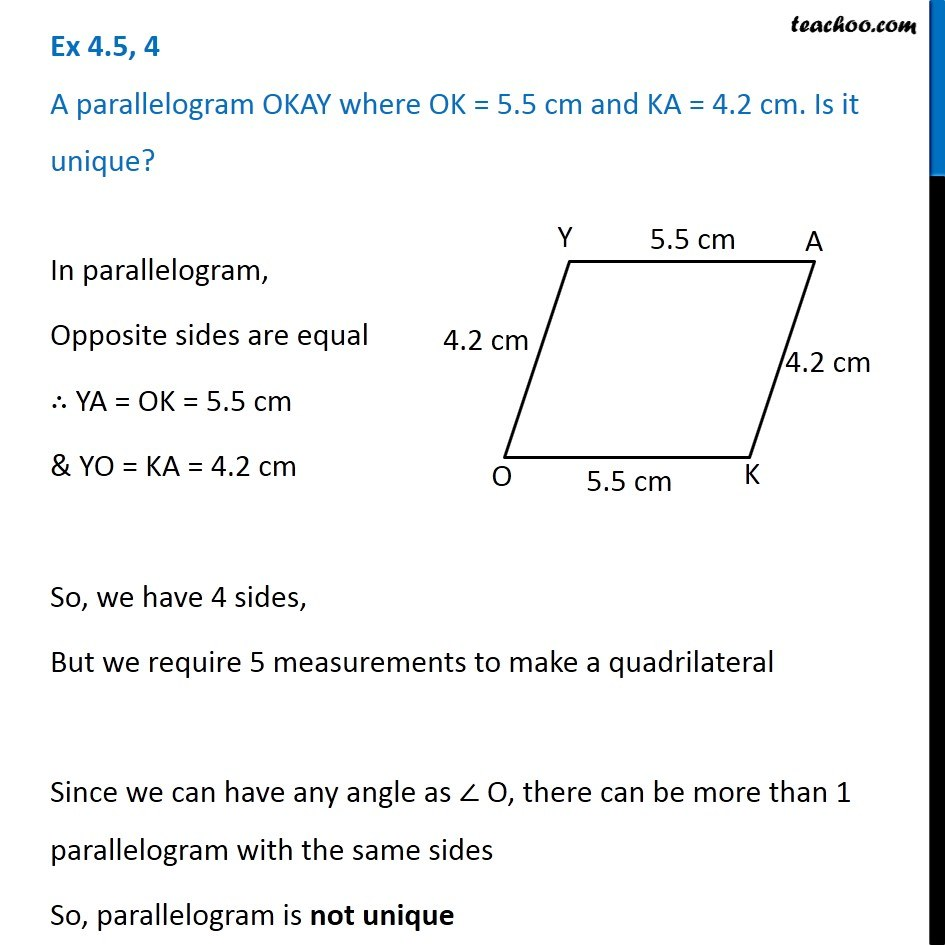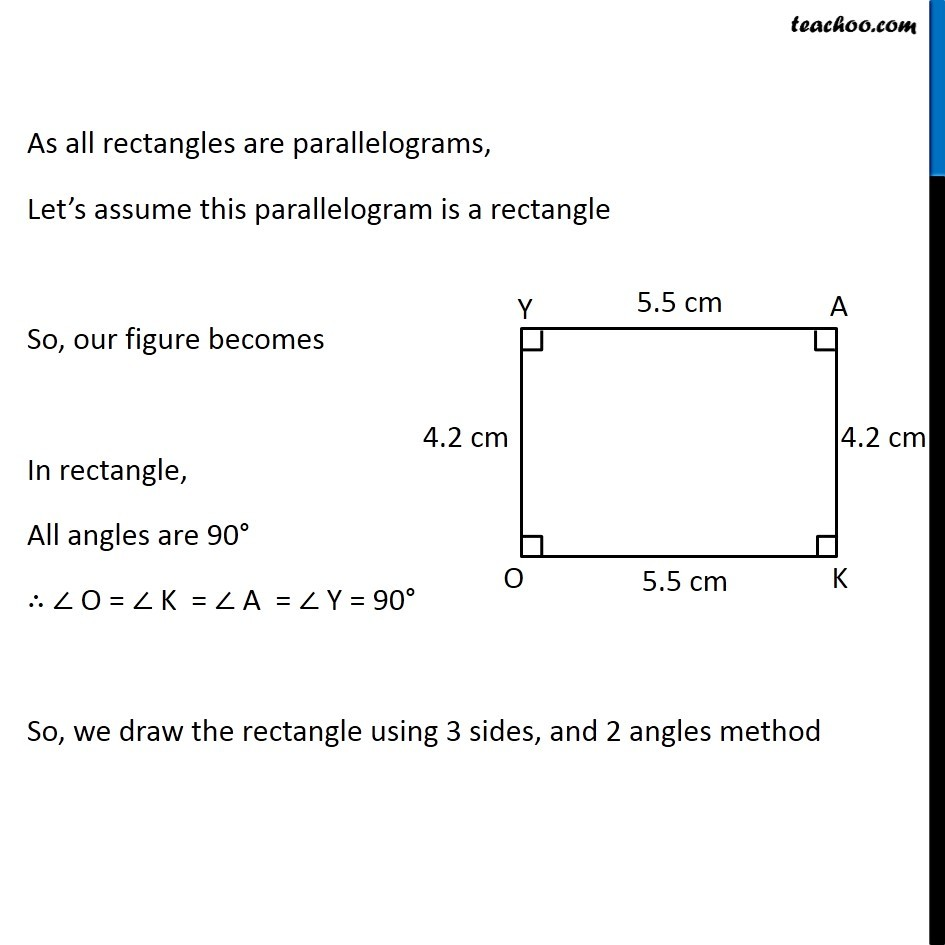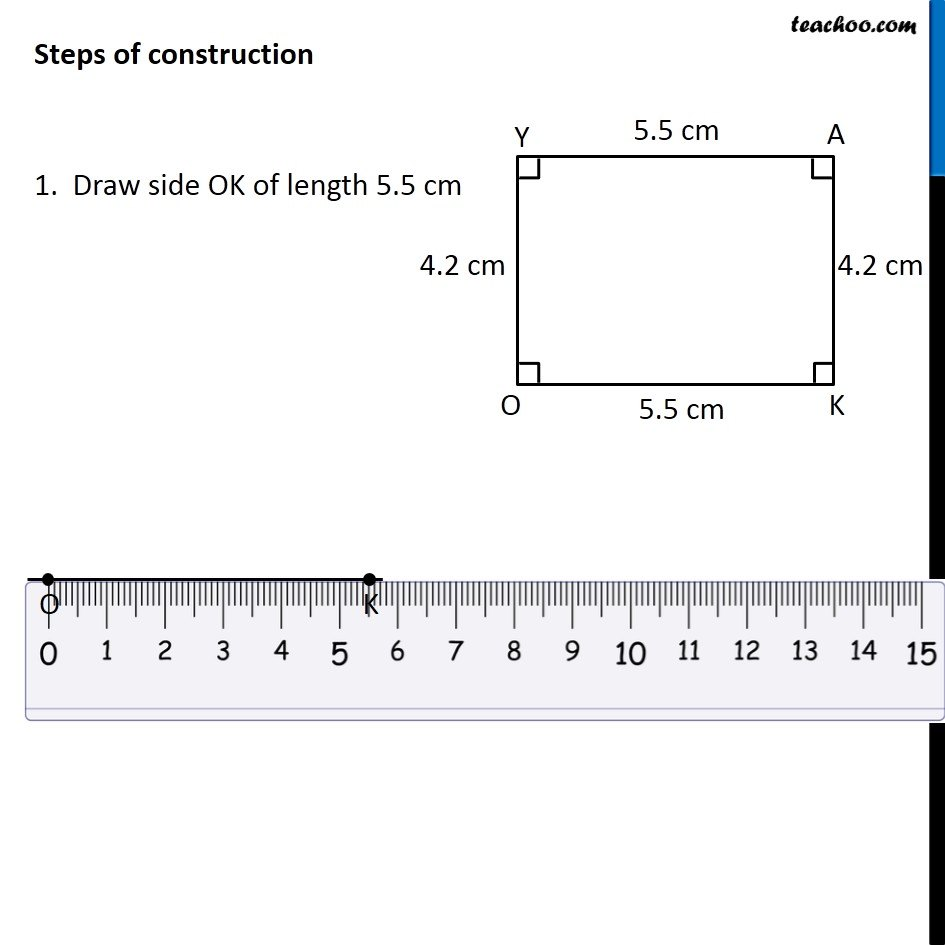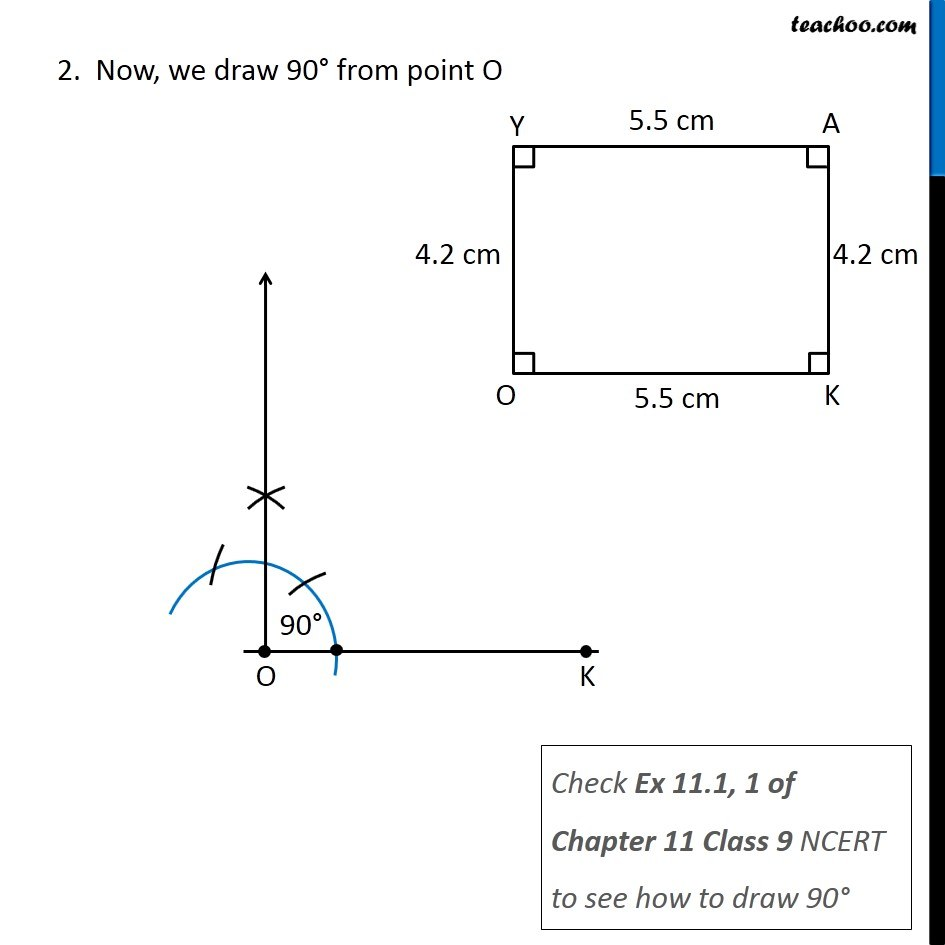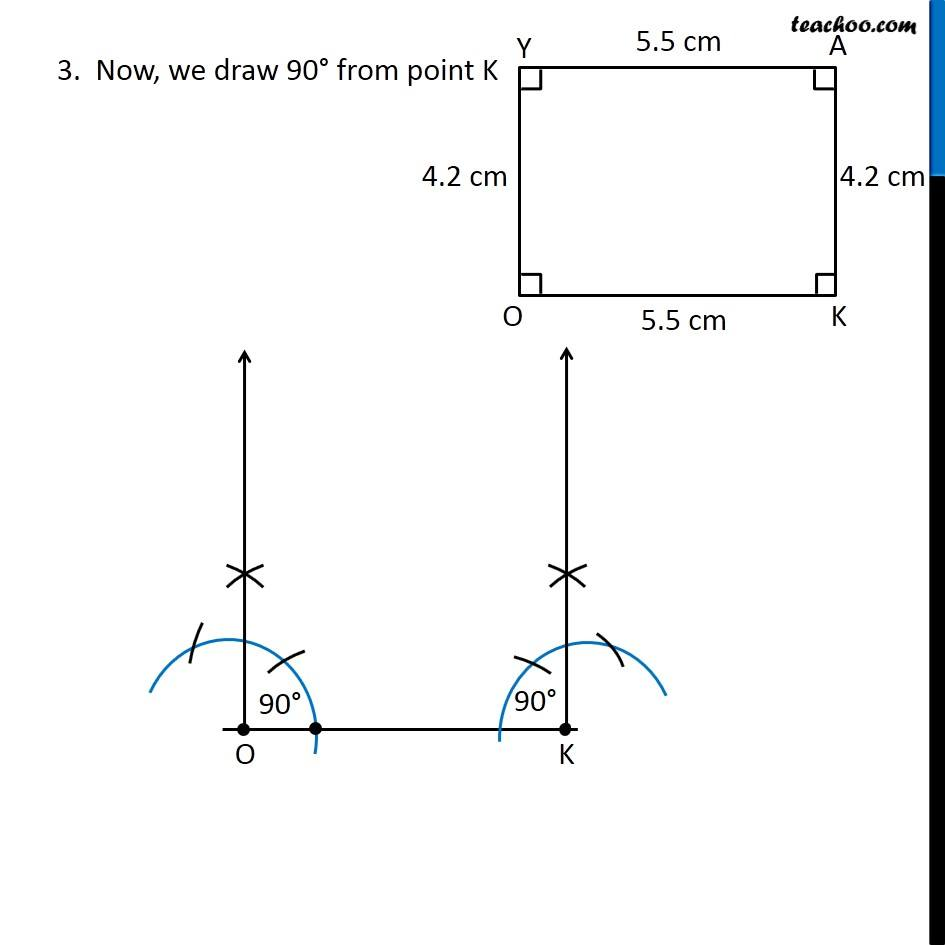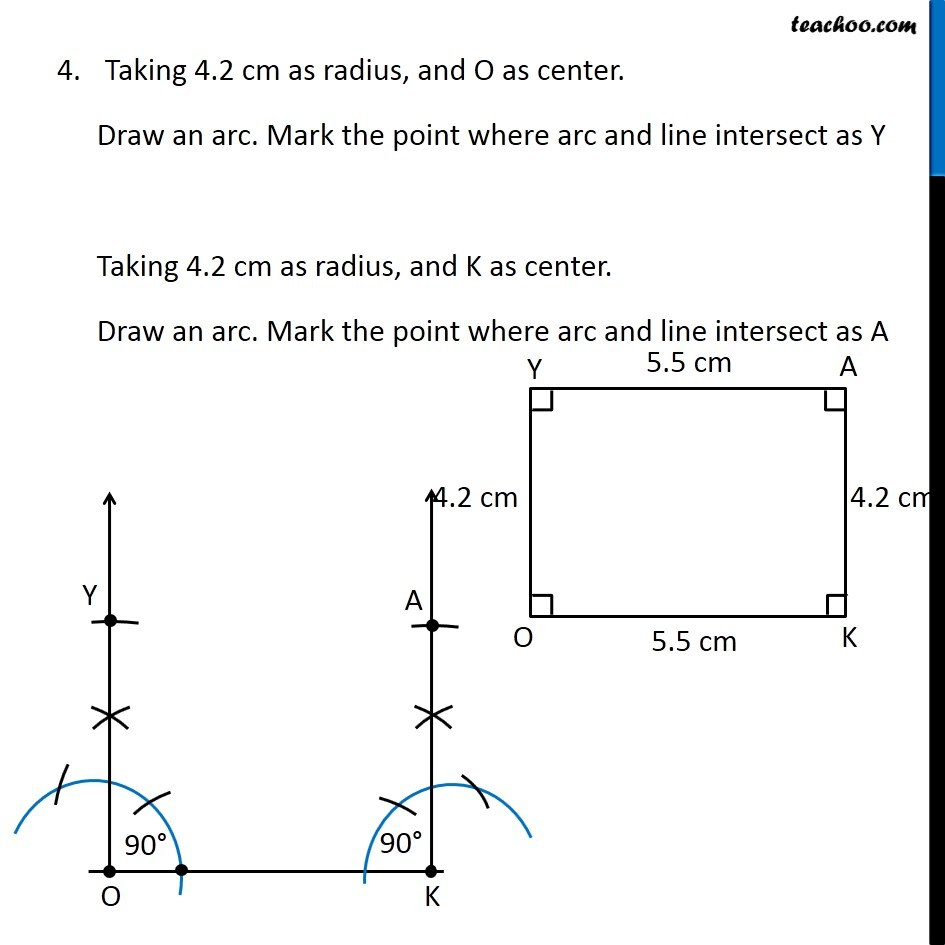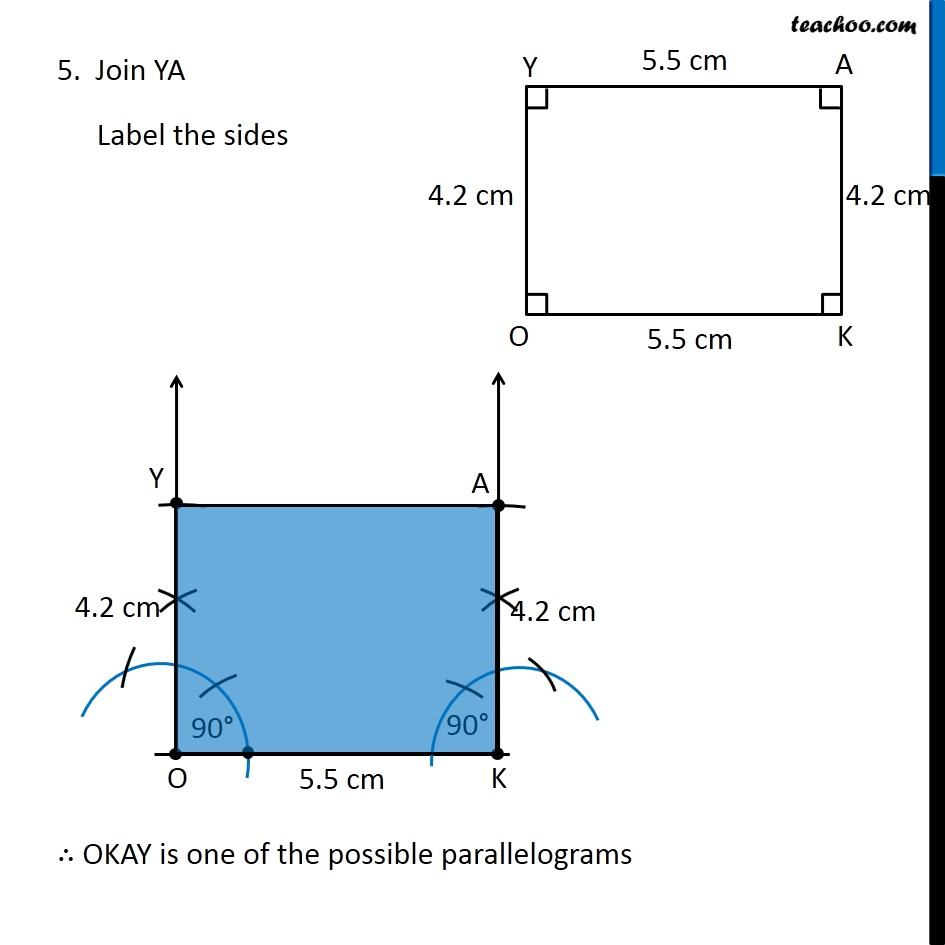Learn in your speed, with individual attention - Teachoo Maths 1-on-1 Class

### Transcript

Question 4 A parallelogram OKAY where OK = 5.5 cm and KA = 4.2 cm. Is it unique? In parallelogram, Opposite sides are equal ∴ YA = OK = 5.5 cm & YO = KA = 4.2 cm So, we have 4 sides, But we require 5 measurements to make a quadrilateral Since we can have any angle as ∠ O, there can be more than 1 parallelogram with the same sides So, parallelogram is not unique In parallelogram, Opposite sides are equal ∴ YA = OK = 5.5 cm & YO = KA = 4.2 cm So, we have 4 sides, But we require 5 measurements to make a quadrilateral Since we can have any angle as ∠ O, there can be more than 1 parallelogram with the same sides So, parallelogram is not unique Steps of construction 1. Draw side OK of length 5.5 cm 2. Now, we draw 90° from point O Check Ex 11.1, 1 of Chapter 11 Class 9 NCERT to see how to draw 90° 3. Now, we draw 90° from point K Taking 4.2 cm as radius, and O as center. Draw an arc. Mark the point where arc and line intersect as Y Taking 4.2 cm as radius, and K as center. Draw an arc. Mark the point where arc and line intersect as A 5. Join YA Label the sides ∴ OKAY is one of the possible parallelograms